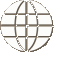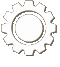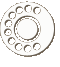COURSE Material
Course picture not available.
Chemistry 30 (Grade 12)

Chemistry 30, or Grade 12 Chemistry, was developed to satisfy the objectives of Saskatchewan Learning for Chemistry 30, and to prepare the student for Saskatchewan’s Chemistry 30 Provincial Departmental Exam. It is written from a Biblical perspective, and is divided into eight units.

The required textbook for this course is Basic Chemistry for Christian Schools by John S. Wetzel, published by Bob Jones University Press. This workbook series was developed for use by schools using an individualized, mastery-based learning system, and therefore comes with score keys, tests, and test keys.

Upon completion of each unit, the student should be able to:

Unit 1

• define matter
• understand the difference between physical and chemical properties of matter and the difference between physical and chemical changes in matter
• classify matter (elements, compounds, and mixtures)
• discuss the states of matter
• use SI units and prefixes
• convert a measurement to a different unit through unit analysis
• apply the rules of significant figures in calculations
• use scientific notation
• identify protons, neutrons, and electrons as components of an atom
• express isotopes using isotopic notation
• calculate atomic mass values when given the percentage of each isotope of an element
• understand and use the periodic table
• determine the molecular mass/formula mass of a given compound
• understand the differences between ionic, covalent, and metallic bonding
• describe the physical properties of ionic, covalent, and metallic compounds
• understand the concept of a mole
• use Avogadro’s number to relate atomic mass units to grams
• determine the molar mass of a given compound
• use the molar mass of a compound to convert between moles and grams

Unit 2

• assign oxidation numbers to compounds
• use oxidation numbers to determine the formula of the compound that results when elements/ions combine
• write the formula of a compound, given its name
• write the name of a compound, given the formula
• balance chemical equations for mass and for charge
• write full ionic and net ionic equations
• apply principles of stoichiometry to chemical equations

Unit 3

• express the concentration of a solution in moles of solute per litre of solution
• manipulate the relationship which links the mass of solute, volume of solution, and concentration of solution, so that given two, the other can be determined
• manipulate the relationship which links original concentration, volume of diluent, and concentration of diluted solution, so that given two, the other may be determined
• relate concentrations expressed as ppm or ppb to those expressed as mol x L-1
• use solubility charts to determine the solubility of various substances
• describe how to perform tests on solutions to determine which ions or ion groups are present
• describe how to separate ions in solution by selective precipitation

Unit 4

• recognize that energy changes are associated with chemical reactions
• relate enthalpy change in a reaction to bond energy and stability
• differentiate between endothermic and exothermic reactions
• compare the energy changes in phase changes and chemical reactions
• explain the difference between heat and temperature
• measure energy changes in chemical reactions
• investigate how tables of standard heats of formation are created and used
• express the enthalpy change of a chemical reaction as a term in the equation for the reactions, or as a heat of reaction
• use tables, graphs, or diagrams and an application of Hess’s Law to infer enthalpy changes in reactions

Unit 5

• identify some factors which affect the rate of chemical reactions
• apply the collision theory to account for the factors which affect the rates of chemical reactions
• recognize that chemical reactions may occur in successive elementary steps
• understand how a series of simple reactions can constitute a reaction mechanism for a complex reaction
• consider molecular level events in a chemical reaction
• discuss the concept of threshold energy
• interpret energy versus reaction pathway diagrams
• consider the existence of transition states in which activated complexes exist
• explain the role of catalysts in chemical reactions
• interpret energy versus reaction pathway diagrams for catalyzed and uncatalyzed reactions

Unit 6

• consider the implications for a system when the rates of the forward and the reverse reactions that define the system are equal
• discuss non-chemical analogies which illustrate or simulate equilibria
• distinguish between dynamic equilibria and steady-state processes
• understand why LeChatelier’s principle works
• use LeChatelier’s principle to predict how various equilibrium systems will shift
• describe how the common ion effect influences the solubility of a solute
• write the equilibrium constant expression for a chemical reaction using the general equation
• analyze graphs of the concentrations of reactants and products with respect to time in a chemical reaction which is approaching equilibrium
• interpret Keq values to determine whether products or reactants are favoured once equilibrium has been reached
• solve problems involving the equilibrium constant expression for a chemical reaction, with concentrations expressed in mol x L-1
• recognize that Keq values are dependent upon temperature but are independent of concentration

Unit 7

• observe some physical and chemical characteristics of acids and bases
• construct an operational definition of an acid and a base
• describe the Bronsted-Lowry conceptual definition of acids and bases
• identify the conjugate bases formed in acid dissociation
• recognize substances which are amphiprotic
• compare the strengths of the dissociations in the dissociation series for a polyprotic acid
• write the equilibrium constant expression for the dissociation of water
• explain how a logarithm scale differs from an arithmetic scale
• estimate the PH of solutions
• state the general neutralization equation
• write equations for specific neutralization reactions
• solve mathematical problems involving data from titrations
• use information from Ka tables to calculate pH values in solutions

Unit 8

• define oxidation and reduction in terms of transfer of electrons
• write half reactions and net ionic equations involving oxidation-reduction
• use a table to compare reduction potentials of half-reactions
• identify and investigate means of protecting metals against corrosion
• determine the direction of electron flow in an electrochemical cell
• measure the voltage in several electrochemical cells
• calculate the potential difference in volts of electrochemical cells, using a standard reduction potential table
• examine applications of electrochemistry
• distinguish between an electrochemical cell and an electrolytic cell
COURSE MaterialsSAICS has developed Christian curriculum (where none other was available) to match Saskatchewan Learning objectives for high school courses.

View Our Course Listing
ORDERING CoursesFind out more information about ordering courses that have been developed by SAICS including score keys and associated textbooks.

Ordering Information